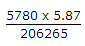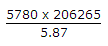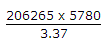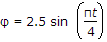# Civil Engineering - UPSC Civil Service Exam Questions

1.
Which one of the following closely represents the shape of the earth?
Spheroid
Ellipsoid
Oblate spheroid
Prolate spheroid
Explanation:
No answer description is available. Let's discuss.

2.
In an old map, line PQ was drawn to a magnetic bearing of 6°32', the magnetic declination at that time being 1° East. The present magnetic declination is 9°42' East. The magnetic bearing to which the line is set at present is
357° 50'
356° 50'
3° 10'
2° 10'
Explanation:
No answer description is available. Let's discuss.

3.
Based on the Gaussian law of distribution of random errors, the number of errors that are expected to exceed the limit of ± a, where 0 represents the standard deviation, out of 1000 observations is
317
46
30
3
Explanation:
No answer description is available. Let's discuss.

4.
In a trigonometric levelling, the height of the signal used is 4.62 m and the instrument height is 1.25 m. If the horizontal distance of the target from the instrument is 5780 m, then the axis-signal correction issecondssecondssecondsseconds
The rotation of a rigid body is governed byWhat is its angular velocity at t - 2s ?